# Important Terms in Hierarchical Clustering

Suppose there are (a) original observations a,…,a[|a|−1] in cluster (a) and (b) original objects b,…,b[|b|−1] in cluster (b), then in order to combine these clusters we need to calculate the distance between two clusters (a) and (b). Say a point (d) exists that hasn’t been allocated to any of the clusters, we need to compute the distance between cluster (a) to (d) and between cluster (b) to (d).

Now clusters usually have multiple points in them that require a different approach for the distance matrix calculation. Linkage decides how the distance between clusters, or point to cluster distance is computed. Commonly used linkage mechanisms are outlined below:

1. Single Linkage — Distances between the most similar members for each pair of clusters are calculated and then clusters are merged based on the shortest distance
2. Average Linkage — Distance between all members of one cluster is calculated to all other members in a different cluster. The average of these distances is then utilized to decide which clusters will merge
3. Complete Linkage — Distances between the most dissimilar members for each pair of clusters are calculated and then clusters are merged based on the shortest distance
4. Median Linkage — Similar to the average linkage, but instead of using the average distance, we utilize the median distance
5. Ward Linkage — Uses the analysis of variance method to determine the distance between clusters
6. Centroid Linkage — Calculates the centroid of each cluster by taking the average of all points assigned to the cluster and then calculates the distance to other clusters using this centroid

These formulas for distance calculation is illustrated in Figure 1 below.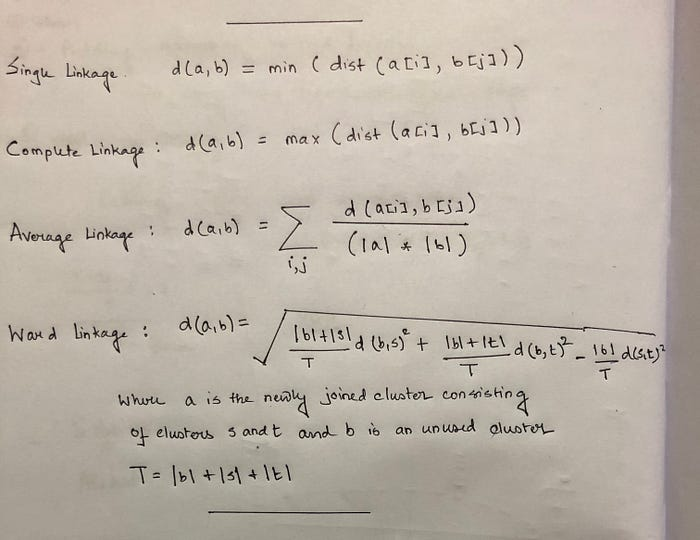Figure 1. Distance formulas for Linkages mentioned above. Image Credit — Developed by the Author

## Distance Calculation

Distance between two or more clusters can be calculated using multiple approaches, the most popular being Euclidean Distance. However, other distance metrics like Minkowski, City Block, Hamming, Jaccard, Chebyshev, etc. can also be used with hierarchical clustering. Figure 2 below outlines how hierarchical clustering is influenced by different distance metrics.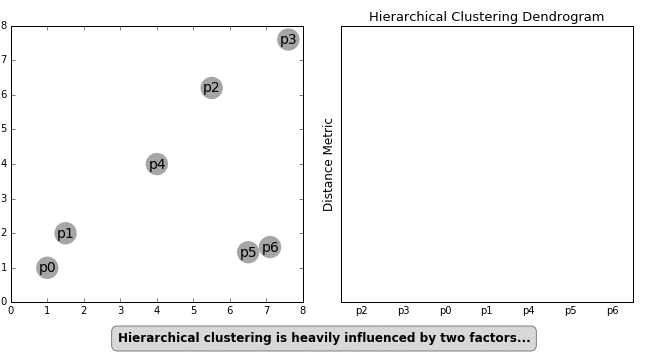Figure 2. Impact of distance calculation and linkage on cluster formation. Image Credits: Image credit — GIF via Gfycat.

## Dendrogram

A dendrogram is used to represent the relationship between objects in a feature space. It is used to display the distance between each pair of sequentially merged objects in a feature space. Dendrograms are commonly used in studying the hierarchical clusters before deciding the number of clusters appropriate to the dataset. The distance at which two clusters combine is referred to as the dendrogram distance. The dendrogram distance is a measure of if two or more clusters are disjoint or can be combined to form one cluster together.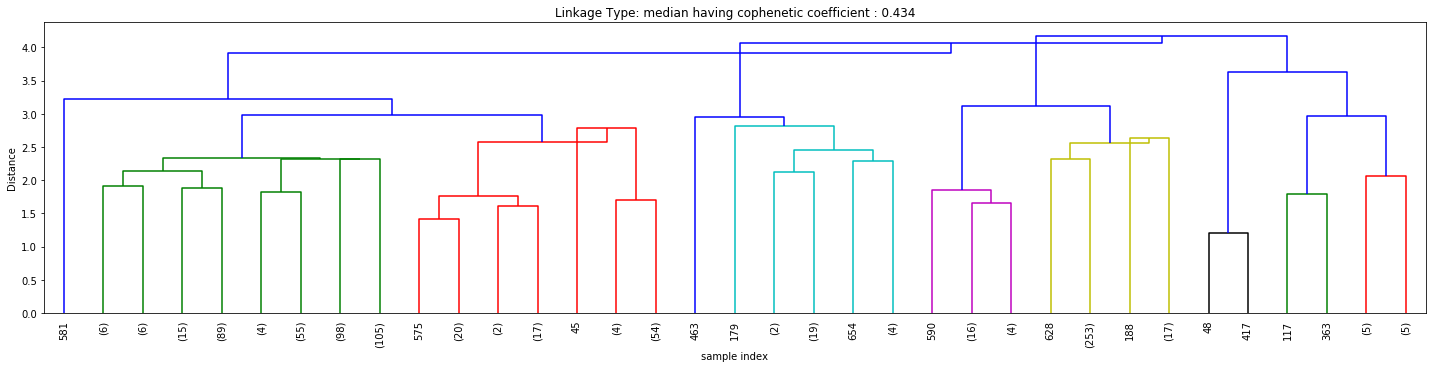Figure 3. Dendrogram a hierarchical clustering using Median as the Linkage Type. Image Credits — Developed by the Author using Jupyter Notebook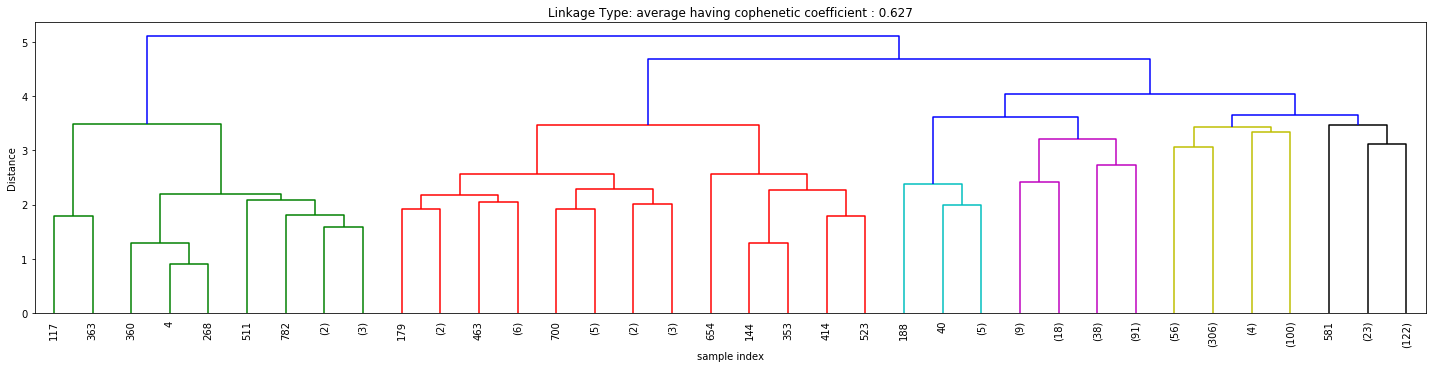Figure 4. Dendrogram of a hierarchical clustering using Average as the Linkage Type. Image Credits — Developed by the Author using Jupyter Notebook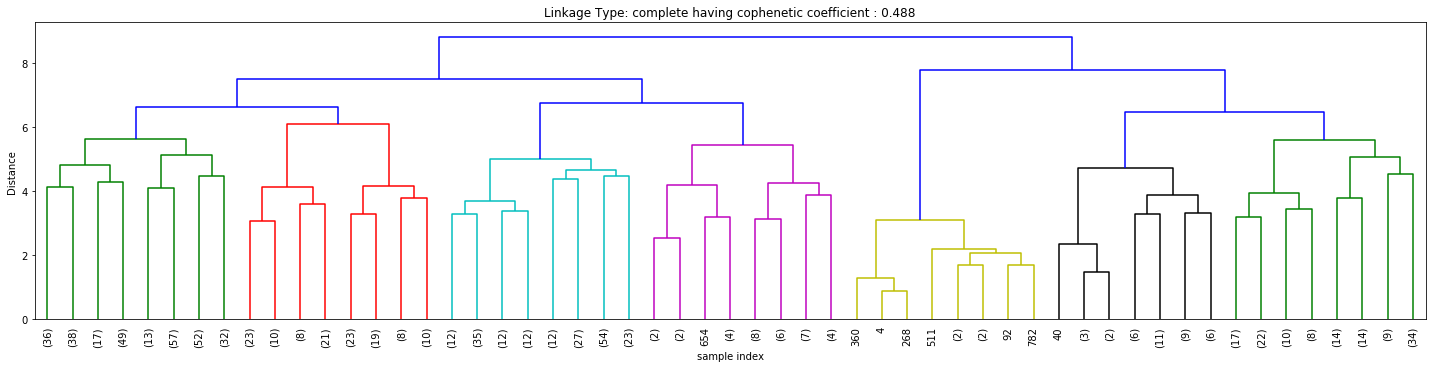Figure 5. Dendrogram of a hierarchical clustering using Complete as the Linkage Type. Image Credits — Developed by the Author using Jupyter Notebook

## Cophenetic Coefficient

Figures 3, 4, and 5 above signify how the choice of linkage impacts the cluster formation. Visually looking into every dendrogram to determine which clustering linkage works best is challenging and requires a lot of manual effort. To overcome this we introduce the concept of Cophenetic Coefficient.

Imagine two Clusters, A and B with points A₁, A₂, and A₃ in Cluster A and points B₁, B₂, and B₃ in cluster B. Now for these two clusters to be well-separated points A₁, A₂, and A₃ and points B₁, B₂, and B₃ should be far from each other as well. Cophenet index is a measure of the correlation between the distance of points in feature space and distance on the dendrogram. It usually takes all possible pairs of points in the data and calculates the euclidean distance between the points. (remains the same, irrespective of which linkage algorithm we chose). It then computes the dendrogram distance at which clusters A & B combines. If the distance between these points increases with the dendrogram distance between the clusters then the Cophenet index is closer to 1.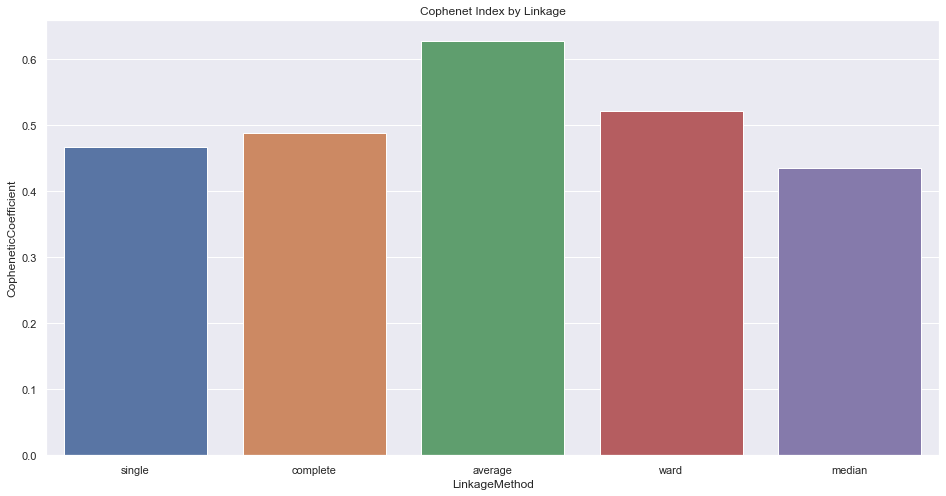Figure 6. Cophenet index of different Linkage Methods in hierarchical clustering. Image Credits — Developed by the Author using Jupyter Notebook

# Deciding the Number of Clusters

There are no statistical techniques to decide the number of clusters in hierarchical clustering, unlike a K Means algorithm that uses an elbow plot to determine the number of clusters. However, one common approach is to analyze the dendrogram and look for groups that combine at a higher dendrogram distance. Let’s take a look at the example below.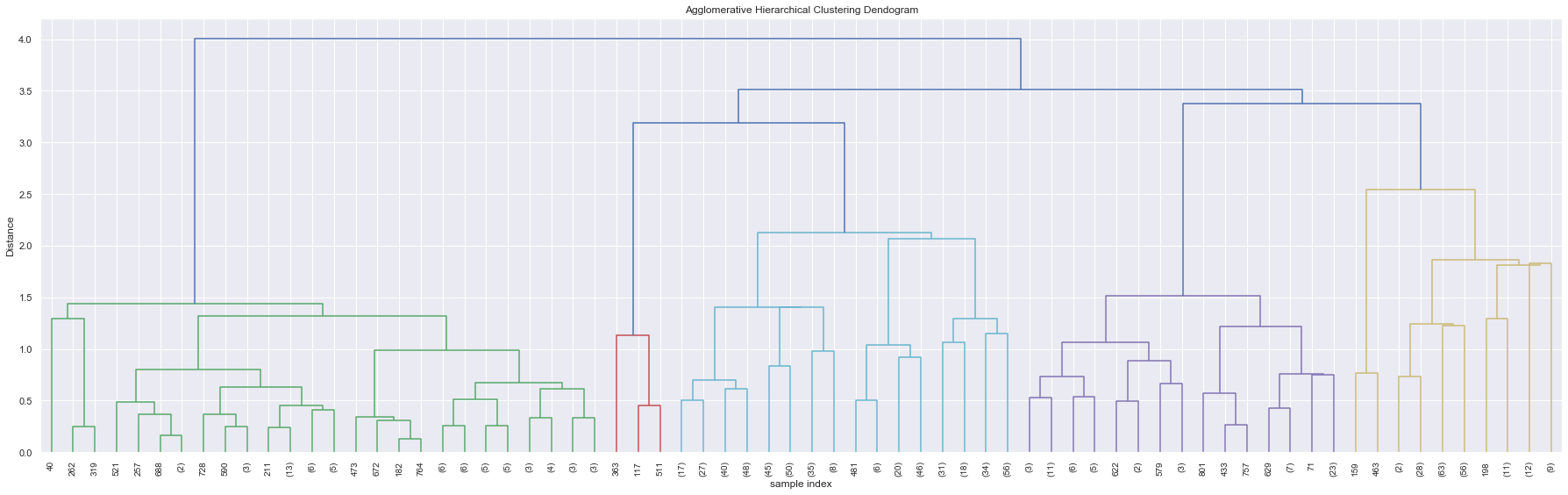Figure 7. Dendrogram of hierarchical clustering using the average linkage method. Image Credits — Developed by the Author using Jupyter Notebook

Figure 7 illustrates the presence of 5 clusters when the tree is cut at a Dendrogram distance of 3. The general idea being, all 5 groups of clusters combines at a much higher dendrogram distance and hence can be treated as individual groups for this analysis. We can also verify the same using a silhouette index score.

# Conclusion

Deciding the number of clusters in any clustering exercise is a tedious task. Since the commercial side of the business is more focused on getting some meaning out of these groups, it is important to visualize the clusters in a two-dimensional space and check if they are distinct from each other. This can be achieved via PCA or Factor Analysis. This is a widely used mechanism to present the final results to different stakeholders that makes it easier for everyone to consume the output.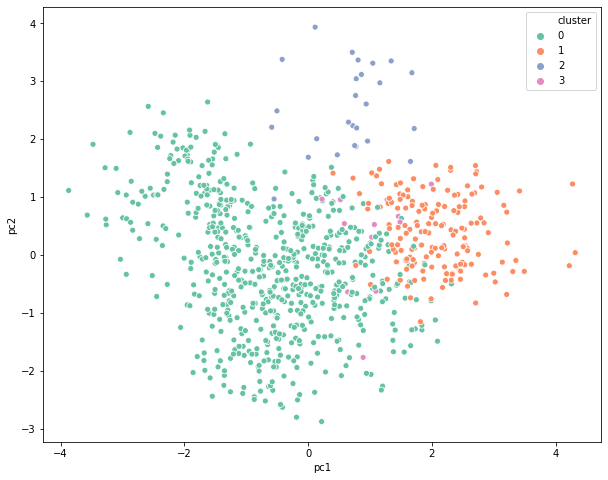Figure 8. Cluster visual of a hierarchical clustering using two different linkage techniques. Image Credits — Developed by the Author using Jupyter Notebook